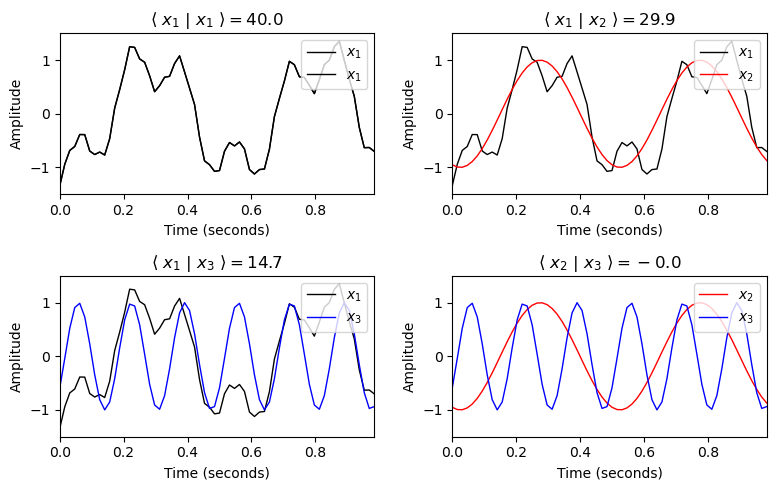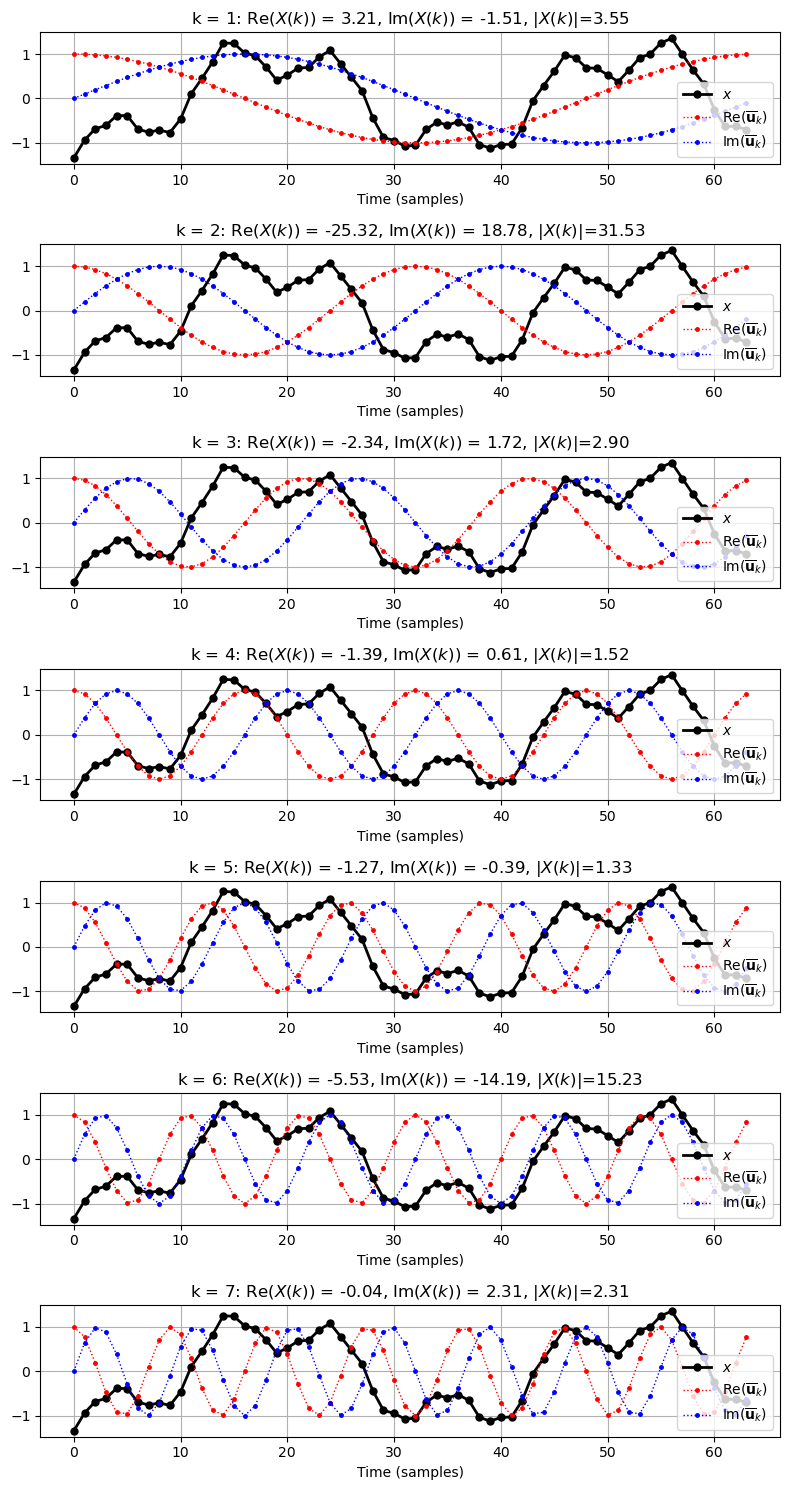# Unit 9: Discrete Fourier Transform (DFT)¶

## Overview and Learning Objectives

The Fourier transform is one of the most important tools for a wide range of engineering and computer science applications. The general idea of Fourier analysis is to decompose a given signal into a weighted superposition of sinusoidal functions. Since these functions possess an explicit physical meaning regarding their frequencies, the decomposition is typically more accessible for subsequent processing steps than the original signal. Assuming that you are familiar with the Fourier transform and its applications in signal processing, we review in this unit the discrete variant of the Fourier transform known as Discrete Fourier Transform (DFT). We define the inner product that allows for comparing two vectors (e.g., discrete-time signals of finite length). The DFT can be thought of as comparing a given signal of finite length with a specific set of exponential signals (a complex variant of sinusoidal signals), each comparison yielding a complex-valued Fourier coefficient. Then, using suitable visualizations, we show how you can interpret the amplitudes and phases of these coefficients. Recall that one can express the DFT as a complex-valued square matrix. We show how separately plotting the real and imaginary parts leads to beautiful and insightful images. Applying a DFT boils down to computing a matrix–vector product, which we implement via the standard NumPy function np.dot. Since the number of operations for computing a DFT via a simple matrix–vector product is quadratic in the input length, the runtime of this approach becomes problematic with increasing length. This issue is exactly where the fast Fourier transform (FFT) comes into the game. We present this famous divide-and-conquer algorithm and provide a Python implementation. Furthermore, we compare the runtime behavior between the FFT implementation and the naive DFT implementation. We will further deepen your understanding of the Fourier transform by considering further examples and visualization in the exercises. In Exercise 1, you will learn how to interpret and plot frequency indices in a physically meaningful way. In Exercise 2, we discuss the issue of loosing time information when applying the Fourier transform, which is the main motivation for the short-time Fourier transform. In Exercise 3, you will apply the DFT to a chirp signal, which yields another illustrative example of the DFT's properties. Finally, in Exercise 4, we will invite you to explore the relationship between the DFT and its inverse. Again, an overarching goal of this unit is to apply and deepen your Python programming skills within the context of a central topic for signal processing.

## Inner Product¶

In this notebook, we consider discrete-time (DT) signals of finite length $N\in\mathbb{N}$, which we represent as vector

$$x=(x(0),x(1),...,x(N-1))^\top\in\mathbb{R}^N$$

with samples $x(n)\in\mathbb{R}^N$ for $n\in[0:N-1]$. Note that $\top$ indicates the transpose of a vector, thus converting a row vector into a column vector. Furthermore, note that we start indexing with the index $0$ (thus adapting our mathematical notation to Python conventions). A general concept for comparing two vectors (or signals) is the inner product. Given two vectors $x, y \in \mathbb{R}^N$, the inner product between $x$ and $y$ is defined as follows:

$$\langle x | y \rangle := \sum_{n=0}^{N-1} x(n) y(n).$$

The absolute value of the inner product may be interpreted as a measure of similarity between $x$ and $y$. If $x$ and $y$ are similar (i.e., if they point to more or less the same direction), the inner product $|\langle x | y \rangle|$ is large. If $x$ and $y$ are dissimilar (i.e., if $x$ and $y$ are more or less orthogonal to each other), the inner product $|\langle x | y \rangle|$ is close to zero.

One can extend this concept to complex-valued vectors $x,y\in\mathrm{C}^N$, where the inner product is defined as

$$\langle x | y \rangle := \sum_{n=0}^{N-1} x(n) \overline{y(n)}.$$

In the case of real-valued signals, the complex conjugate does not play any role and the definition of the complex-valued inner product reduces to the real-valued one. In the following code cell, we give some examples.

Note: One can use the NumPy function np.vdot to compute the inner product. However, opposed to the mathematical convention that conjugates the second argument, this function applies complex conjugation on the first argument. Therefore, for computing $\langle x | y \rangle$ as defined above, one has to call np.vdot(y, x).

In the following, we generate and visualize three signals $x_1$, $x_2$, $x_3$. Then, we compute and discuss different inner products using the signals.

In :
import numpy as np
from matplotlib import pyplot as plt
import libpcp.signal
%matplotlib inline

Fs = 64
dur = 1
x1, t = libpcp.signal.generate_example_signal(Fs=Fs, dur=dur)
x2, t = libpcp.signal.generate_sinusoid(dur=dur, Fs=Fs, amp=1, freq=2, phase=0.3)
x3, t = libpcp.signal.generate_sinusoid(dur=dur, Fs=Fs, amp=1, freq=6, phase=0.1)

def plot_inner_product(ax, t, x, y, color_x='k', color_y='r', label_x='x', label_y='y'):
"""Plot inner product

Notebook: PCP_09_dft.ipynb

Args:
ax: Axis handle
t: Time axis
x: Signal x
y: Signal y
color_x: Color of signal x (Default value = 'k')
color_y: Color of signal y (Default value = 'r')
label_x: Label of signal x (Default value = 'x')
label_y: Label of signal y (Default value = 'y')
"""
ax.plot(t, x, color=color_x, linewidth=1.0, linestyle='-', label=label_x)
ax.plot(t, y, color=color_y, linewidth=1.0, linestyle='-', label=label_y)
ax.set_xlim([0, t[-1]])
ax.set_ylim([-1.5, 1.5])
ax.set_xlabel('Time (seconds)')
ax.set_ylabel('Amplitude')
sim = np.vdot(y, x)
ax.set_title(r'$\langle$ %s $|$ %s $\rangle = %.1f$' % (label_x, label_y, sim))
ax.legend(loc='upper right')

plt.figure(figsize=(8, 5))
ax = plt.subplot(2, 2, 1)
plot_inner_product(ax, t, x1, x1, color_x='k', color_y='k', label_x='$x_1$', label_y='$x_1$')
ax = plt.subplot(2, 2, 2)
plot_inner_product(ax, t, x1, x2, color_x='k', color_y='r', label_x='$x_1$', label_y='$x_2$')
ax = plt.subplot(2, 2, 3)
plot_inner_product(ax, t, x1, x3, color_x='k', color_y='b', label_x='$x_1$', label_y='$x_3$')
ax = plt.subplot(2, 2, 4)
plot_inner_product(ax, t, x2, x3, color_x='r', color_y='b', label_x='$x_2$', label_y='$x_3$')
plt.tight_layout()In the above example, one can make the following observations:

• The signal $x_1$ is similar to itself, leading to a large value of $\langle x_1 | x_1 \rangle=40.0$.
• The overall course of the signal $x_1$ strongly correlates with the sinusoid $x_2$, which is reflected by a relatively large value of $\langle x_1 | x_2 \rangle=29.9$.
• There are some finer oscillations of $x_1$ that are captured by $x_3$, leading to a still noticeable value of $\langle x_1 | x_3 \rangle=14.7$.
• The two sinusoids $x_2$ and $x_3$ are more or less uncorrelated, which is revealed by the value of $\langle x_2 | x_3 \rangle\approx 0$.

In other words, the above comparison reveals that the signal $x_1$ has a strong signal component of $2~\mathrm {Hz}$ (frequency of $x_2$) and $6~\mathrm {Hz}$ (frequency of $x_3$). Measuring correlations between an arbitrary signal and sinusoids of different frequencies is exactly the idea of performing a Fourier (or spectral) analysis.

## Definition of DFT¶

Let $x\in \mathbb{C}^N$ be a vector of length $N\in\mathbb{N}$. The discrete Fourier transform (DFT) of $x$ is defined by:

$$X(k) := \sum_{n=0}^{N-1} x(n) \exp(-2 \pi i k n / N)$$

for $k \in [0:N-1]$. The vector $X\in\mathbb{C}^N$ can be interpreted as a frequency representation of the time-domain signal $x$. To obtain a geometric interpretation of the DFT, we define the vector $\mathbf{e}_k \in\mathbb{C}^N$ with real part $\mathbf{c}_k=\mathrm{Re}(\mathbf{e}_k)$ and imaginary part $\mathbf{s}_k=\mathrm{Im}(\mathbf{e}_k)$ by

$$\mathbf{e}_k(n) := \exp(2 \pi i k n / N) = \cos(2 \pi i k n / N) + i \sin(2 \pi i k n / N) = \mathbf{c}_k(n) + i \mathbf{s}_k(n)$$

for each $k \in [0:N-1]$.

This vector can be regarded as a sampled version of the exponential function of frequency $k/N$. Using inner products, the DFT can be expressed as

$$X(k) = \sum_{n=0}^{N-1} x(n) \overline{\mathbf{e}_k}(n) = \langle x | \mathbf{e}_k \rangle,$$

thus measuring the similarity between the signal $x$ and the sampled exponential functions $\mathbf{e}_k$. The absolute value $|X(k)|$ indicates the degree of similarity between the signal $x$ and $\mathbf{e}_k$. In the case that $x\in \mathbb{R}^N$ is a real-valued vector (which is typically the case for audio signals), we obtain:

$$X(k) = \langle x |\mathrm{Re}(\mathbf{e}_k) \rangle - i\langle x | \mathrm{Im}(\mathbf{e}_k) \rangle = \langle x |\mathbf{c}_k \rangle - i\langle x | \mathbf{s}_k \rangle$$

The following plot shows an example signal $x$ compared with functions $\overline{\mathbf{e}_k}$ for various frequency parameters $k$. The real and imaginary part of $\overline{\mathbf{e}_k}$ are shown in red and blue, respectively.

In :
def plot_signal_e_k(ax, x, k, show_e=True, show_opt=False):
"""Plot signal and k-th DFT sinusoid

Notebook: PCP_09_dft.ipynb

Args:
ax: Axis handle
x: Signal
k: Index of DFT
show_e: Shows cosine and sine (Default value = True)
show_opt: Shows cosine with optimal phase (Default value = False)
"""
N = len(x)
time_index = np.arange(N)
ax.plot(time_index, x, 'k', marker='.', markersize='10', linewidth=2.0, label='$x$')
plt.xlabel('Time (samples)')
e_k = np.exp(2 * np.pi * 1j * k * time_index / N)
c_k = np.real(e_k)
s_k = np.imag(e_k)
X_k = np.vdot(e_k, x)

plt.title(r'k = %d: Re($X(k)$) = %0.2f, Im($X(k)$) = %0.2f, $|X(k)|$=%0.2f' %
(k, X_k.real, X_k.imag, np.abs(X_k)))
if show_e is True:
ax.plot(time_index, c_k, 'r', marker='.', markersize='5',
linewidth=1.0, linestyle=':', label='$\mathrm{Re}(\overline{\mathbf{u}}_k)$')
ax.plot(time_index, s_k, 'b', marker='.', markersize='5',
linewidth=1.0, linestyle=':', label='$\mathrm{Im}(\overline{\mathbf{u}}_k)$')
if show_opt is True:
phase_k = - np.angle(X_k) / (2 * np.pi)
cos_k_opt = np.cos(2 * np.pi * (k * time_index / N - phase_k))
d_k = np.sum(x * cos_k_opt)
ax.plot(time_index, cos_k_opt, 'g', marker='.', markersize='5',
linewidth=1.0, linestyle=':', label='$\cos_{k, opt}$')
plt.grid()
plt.legend(loc='lower right')

N = 64
x, t = libpcp.signal.generate_example_signal(Fs=N, dur=1)

plt.figure(figsize=(8, 15))
for k in range(1, 8):
ax = plt.subplot(7, 1, k)
plot_signal_e_k(ax, x, k=k)

plt.tight_layout()## DFT Phase¶

At first sight, the DFT may be a bit confusing: Why is a real-valued signal $x$ compared with a complex-valued sinusoid $\mathbf{e}_k$? What does the resulting complex-valued Fourier coefficient

$$c_k:= X(k) := \langle x |\mathrm{Re}(\mathbf{e}_k) \rangle - i\langle x | \mathrm{Im}(\mathbf{e}_k) \rangle.$$

encode? To understand this, we represent the complex number $c_k$ in form of its polar representation

$$c_k = |c_k| \cdot \mathrm{exp}(i \gamma_k),$$

where $\gamma_k$ is the angle (given in radians). Furthermore, let $\mathbf{cos}_{k,\varphi}:[0:N-1]\to\mathbb{R}$ be a sampled sinusoid with frequency parameter $k$ and phase $\varphi\in[0,1)$, defined by

$$\mathbf{cos}_{k,\varphi}(n) = \mathrm{cos}\big( 2\pi (kn/N - \varphi) \big)$$

for $n\in[0,N-1]$. Defining $\varphi_k := - \frac{\gamma_k}{2 \pi}$, one obtains the following remarkable property of the Fourier coefficient $c_k$:

\begin{eqnarray} |c_k| &=& \mathrm{max}_{\varphi\in[0,1)} \langle x | \mathbf{cos}_{k,\varphi} \rangle,\\ \varphi_k &=& \mathrm{argmax}_{\varphi\in[0,1)} \langle x | \mathbf{cos}_{k,\varphi} \rangle. \end{eqnarray}

In other words, the phase $\varphi_k$ maximizes the correlation between $x$ and all possible sinusoids $\mathbf{cos}_{k,\varphi}$ with $\varphi\in[0,1)$. Furthermore, the magnitude $|c_k|$ yields this maximal value. Thus, computing a single correlation between $x$ and the complex-valued function $\mathbf{e}_k$ (which real part coincides with $\mathbf{cos}_{k,0}$, and its imaginary part with $\mathbf{cos}_{k,0.25}$) solves an optimization problem. In the following code cell, we demonstrate this optimality property, where the $\mathbf{cos}_{k,\varphi}$ with optimal phase $\varphi=\varphi_k$ is shown in green.

In :
plt.figure(figsize=(8, 15))
for k in range(1, 8):
ax = plt.subplot(7, 1, k)
plot_signal_e_k(ax, x, k=k, show_e=False, show_opt=True)

plt.tight_layout()